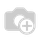# Filter last year and last month

I've created a filter for filter last month and last year of order it doesn't work correctly

``````<filter icon="terp-go-month" string="Last Year"
domain="[('date_order','&lt;=', (context_today()-relativedelta(day=31,months=12,years=1)).strftime('%%Y-%%m-%%d')),
('date_order','&gt;=',(context_today()-relativedelta(day=1,months=1,years=1)).strftime('%%Y-%%m-%%d'))]"
help="last year"/>

<filter icon="terp-go-month" string="Last Month"
domain="[('date_order','&lt;=', (context_today()-relativedelta(day=31, months=1)).strftime('%%Y-%%m-%%d')),
('date_order','&gt;=',(context_today()-relativedelta(day=1,months=1)).strftime('%%Y-%%m-%%d'))]"
help="last month"/>
``````

and I create filter for month like this it works correctly

``````<filter icon="terp-go-month" string="Month"
name="month"
domain="[('date_order','&lt;=',
(context_today()+relativedelta(day=31)).strftime('%%Y-%%m-%%d')),
('date_order','&gt;=',
(context_today()-relativedelta(day=1)).strftime('%%Y-%%m-%%d'))]"
help="current month"/>
``````

I dont know why? Thank for supportThis is a correct way to filter the current month. Use it to create your own filter:

``````<filter string="Current Month" name="current_month" domain="[('data','&lt;',(context_today()+relativedelta(months=1)).strftime('%%Y-%%m-01')), ('data','&gt;=',time.strftime('%%Y-%%m-01'))]"/>
``````

This is an example of previous month:

``````<filter string="Prev Month" name="prev_month" domain="[('data','&gt;=',(context_today()-relativedelta(months=1)).strftime('%%Y-%%m-01')),('data','&lt;',time.strftime('%%Y-%%m-01'))]"/>
``````
1 CommentThis is an example of 'Last Year':



This is an example of 'Last Month':